## Grouped data

To understand a data set with a large number of values, it is helpful both to divide the range of values of the data into intervals, called class intervals or classes, and to record the frequency of each class interval, the number of data items in each class. The resulting summary of the data is called a grouped frequency table or simply a frequency table.

#### Example 3

The marks out of 50 for a mathematics test done by a class of 25 students are:

 48 43 29 36 37 21 15 24 35 44 37 35 25 29 39 28 25 46 37 24 26 42 45 33 47

Present this information in a frequency table, using groupings of 15–19, 20–24, 25–29 and so on up to 45–49.

#### Solution

Grouping the data produces the following frequency table:

Mark Tally Frequency
15–19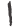1
20–24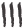3
25–29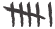6
30–341
35–39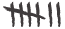7
40–443
45–49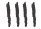4